# Cutting Slip Velocity Calculation Method 2

This is another method to determine cutting slip velocity. The process of calculation is quite different from the first method however it is still straight forward calculation. It still gives you the following answers: annular velocity, cutting slip velocity and net velocity.

Let’s get started with this calculation method.

1. Determine n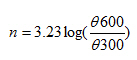Where;

n is the power law exponent.

Θ600 is a value at 600 viscometer dial reading.

Θ300 is a value at 300 viscometer dial reading.

2. Determine K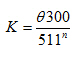Where;

K is the fluid consistency unit

Θ300 is a value at 300 viscometer dial reading.

n is the power law exponent.

3. Determine annular velocity with following equation:Where;

AV is annular velocity in ft/min.

Q is flow rate in gpm (gallon per minute).

Dh is diameter of hole in inch.

Dp is diameter of drill pipe in inch.

4. Determine cutting slip velocity with following equation:Where;

µ is viscosity in centi-poise.

V is annular velocity in ft/min.

Dh is diameter of hole in inch.

Dp is diameter of drill pipe in inch.

n is dimensionless number from the equation 1

K is dimensionless number from the equation 2

5. Slip Velocity (Vs) in ft/min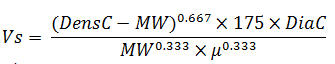Where;

Vs is slip velocity in ft/min.

DensC is cutting density in ppg

DiaC is cutting diameter in inch

µ is viscosity in centi-poise given from equation#4.

6. Determine net rise velocity with following equation:

Net rise velocity = AV – Vs

Where;

AV is annular velocity.

Vs Cutting Velocity

This figure indicates that cuttings are being lifted by mud or are still falling down.

If net rise velocity is positive, it means that you have good flow rate which can carry cuttings in the wellbore.

On the other hand, If net rise velocity is negative, your current flow rate is NOT enough to carry cuttings.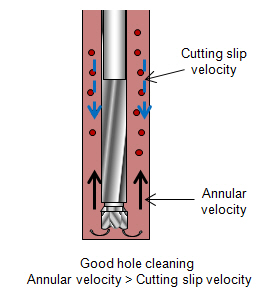Example: Please use the following information to determine annular velocity, cutting slip velocity, net rise velocity, and tell us if the flow rate is good for hole cleaning.

θ300 = 25

θ600 = 40

Flow rate = 750 gpm

Hole size = 12.0 inch

Drill pipe diameter = 5 inch

Cutting diameter = 0.3 inch

Cutting density = 22 ppg

Mud weight = 10 ppg

1. Determine n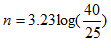n = 0.678

2. Determine K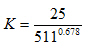K = 0.365

3. Determine annular velocity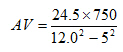AV = 154.4 ft/min

4. Determine cutting slip velocity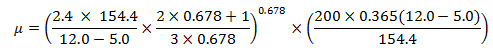µ = 53.88 centi-poise

5. Determine slip velocity (Vs)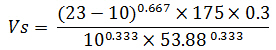Vs = 35.78 ft/min

6. Determine net rise velocity

Net rise velocity = 154.4 – 35.78 = 118.6 ft/min

Conclusion: This flow rate is good for hole cleaning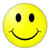because annular velocity is more than cutting slip velocity.

Share the joyWorking in the oil field and loving to share knowledge.

### 4 Responses to Cutting Slip Velocity Calculation Method 2

1.Diganta Chowlu says:

i like your site very much. it give lot helps peoples who were working. drilling rigs.

2.andi says:

what formula to predict cutting bed (% bed & bed height)?

•DrillingFormulas.Com says:

We don’t have the eqatuion to determine a cutting bed.

This site uses Akismet to reduce spam. Learn how your comment data is processed.